# Three-Rail Kicking Systems

Posted by : .(JavaScript must be enabled to view this email address)

Today we're going to show you a couple three-rail kicking systems. The first is a visual system that is VERY easy to use and is what I’d recommend for most players. The second aiming system is more precise for making contact anywhere on the table but is also more complex using a formula and numbers assigned to spots on the table. Both of these systems are great to know and can get you out of some tricky situations!

## Visual System for Three-Rail Kick Shots: Projecting to an Aiming Point in the Distance

The first method is more visual than mathematical; however it works pretty well in some instances. First, you need to find the standard way to scratch [the cue ball] going three rails. We're going to try to scratch in this corner here by going three rails: long rail here, short rail there, long rail there to scratch here. On an imaginary “ideal” pool table, shooting into the third diamond with running English would lead to the scratch we’re looking for. The cue ball would hit the long rail, short rail, long rail, and land straight in the corner. Contrary to popular belief, shooting into the third diamond will not work on most tables. Most pool tables will play “long” or “short”, depending on the construction and condition.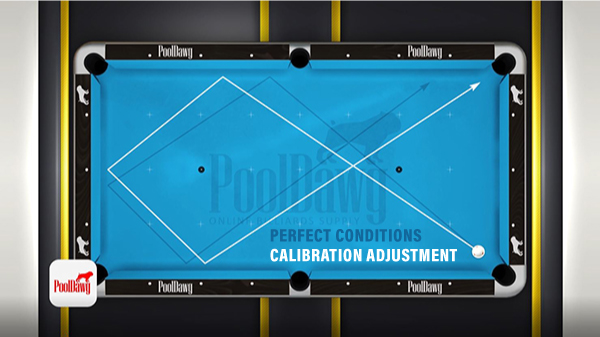So the first thing we're going to do is test the table to see if the table plays long or short. If it plays “short”, the cue ball is going to land above the pocket (long rail). If it plays “long”, the cue ball is going to land below the pocket (end rail). Okay, now Valerie is going to try to scratch, aiming to this diamond here first. See what happens with the cue ball.

Okay, we see the cue ball here is about a full diamond short, if not more. We will need to take that in consideration. She's going to aim higher, probably about the second diamond this time, and we'll see what happens.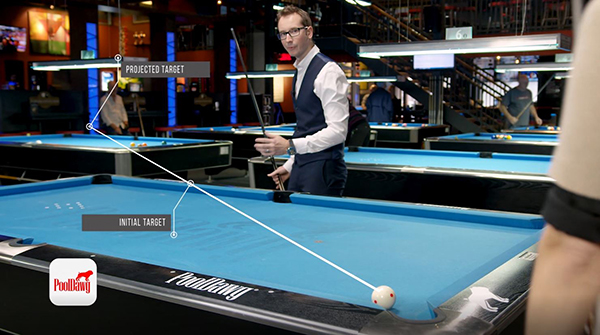It's looking good, and we've got a perfect scratch here. Now that Valerie found a perfect scratching point, we're going to draw a line from that diamond here, wherever she found it, into the center of the ball out there. And we're going to extend that line out to a point in the rest of the room. For best results the point should be about 10 – 20 feet away. Starting from the ball to the pocket, then to the diamond, and out into the room is that neon blue post out there. We’re going to use the left side as our aiming point now. Now, we are not even going to look at the rails, but aim at the neon out there and scratch again.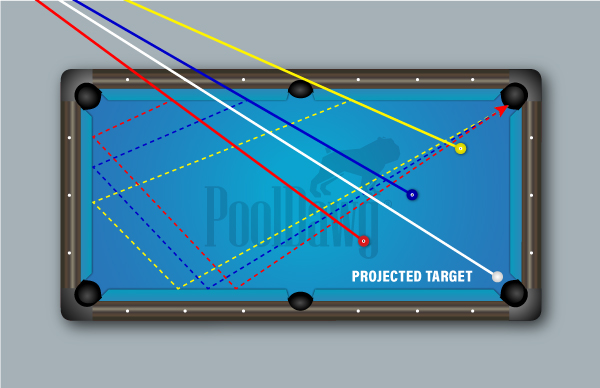Now that you have this spot on the wall, the beauty of this system is that no matter where the cue ball is, you can reuse the point of aim to make the three rail kick. If your cue ball is somewhere here, up to probably about here between the side pocket and the corner there, the same point of aim will work.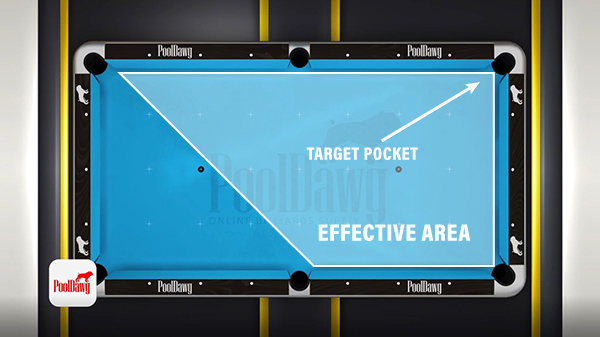Anything after the start of the side pocket to the opposite corner doesn't work because you can't go three rails from there. What we're going to do now is shoot these three balls that pretending are cue balls, the one, the two, and the three, and make the four ball in the corner. Valerie is going to shoot them using the three rail aiming method each time, with the same spot on the wall out there.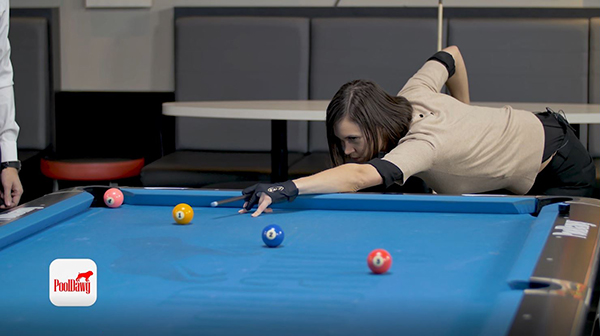Perfect. And that's how you execute a proper three rail by always aiming the same spot on the wall. So as you can see, anywhere the ball was, it worked. Great system. Great visual. As long as you find your spot, you'll always be pretty close to making the three rail kick.

## “Mathematical” Three Rail Kicking System – Using a Formula to Find the Proper Aiming Point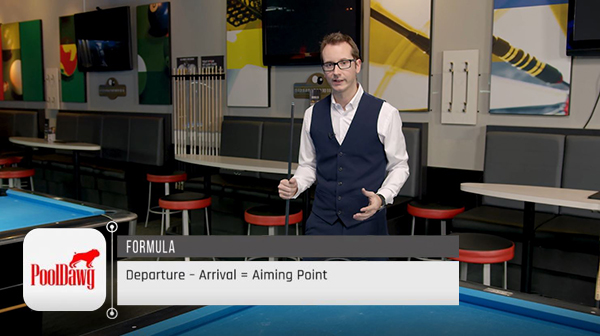Let's talk about the mathematical system now. Before we go any further, a disclaimer; this is a very complex system, very hard to understand, very hard to remember. So it's not uncommon to see even pros that don't know anything about it. So, before you feel bad or you try to really dive deep into it, if you don't play a lot of hours, don't think about it. The visual one will work great for you. That being said, this system is absolutely great, very effective, very accurate, and it works all around the table. So there are pretty much no limits. This is a system we use a lot in three cushion and the best three cushion players all know it and use it everywhere on the table.

Formula: Departure – Arrival = Aiming Point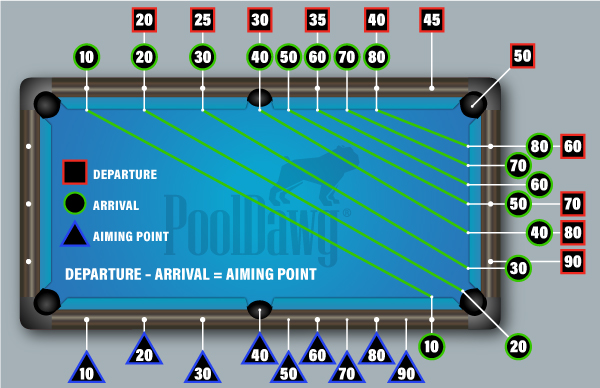So let's start with the system by itself. It's basically composed of a formula. The formula is “departure minus arrival equals aiming points”.

### Departure Values:

The departure values are going to be this way. Before we go any further, this is going to be our shot (ball place in the corner) - long rail here (left side), short rail there (far rail), long rail there (right side) into the one ball. First, I'm going to try to find my departure point. The departure is going to be somewhere from here to there. Personally I use the number 50, but I know some people use the five, which is also why we call this system the “corner five”, but I'm going to call this corner 50. You’re just going to have to remember the value. This is 50.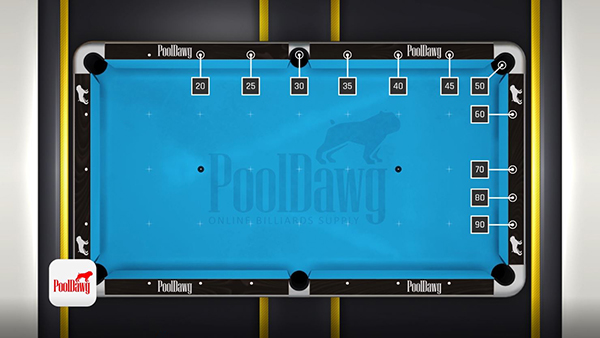It goes 45, 40, 35, 30, 25, 20, and not much is needed out there. So it goes by increments of five up there. Now, this is still the departure rail, however the short rail goes by 10. So this goes 60, 70 here, and now it goes 80, 90. We're not here to really discuss why, but it's basically because the table plays short or longer at some part of the table, depending the rails. Once again, this system works great on three cushion heated table, on many pool table they play short and require adjustments.

### Arrival Values: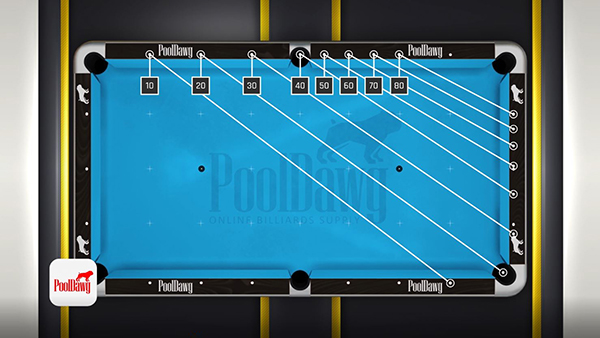Now we have the departure points, we've got to talk about arrival. Arrival is the next part in the formula. Arrival is going to go from the one ball. Now you would think it would go on these rails, however, it does not. The arrival is taken from the last rail the cue ball hits facing the object ball you're trying to hit. So this is our arrival rail. This one goes 10, 20, 30, 40. Because the table plays a little differently, this is going to be 50, 60, 70, 80. Now from there, there's one thing I cannot really teach you, and that is you have to remember the line. So we know that line, the 20 goes here and kind of extend to the corner. The 30 goes here, extend to this diamond. The 10 goes here, extend to this diamond. And technically right next to the pocket, extend to the zero at the second diamond. It goes all the way down there, you can see in the graphic.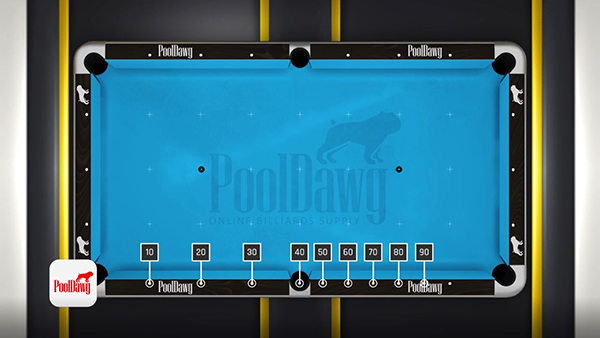Now you've determined your arrival points. We said the cue ball is in 50 because it's in the corner; object ball arrival at 20. The formula is departure minus arrival (50 – 20 = 30). So this is my aiming rail, aiming point as well. It goes by increments of 10. So zero, 10, 20, 30, 40, 50, et cetera, et cetera. Again, we invite you to look at the diagram, it's easier to understand. Departure minus arrival, 50 minus 20, equals 30. In the perfect table in a perfect world with a perfect stroke, aiming at the 30 you are guaranteed to make the one ball.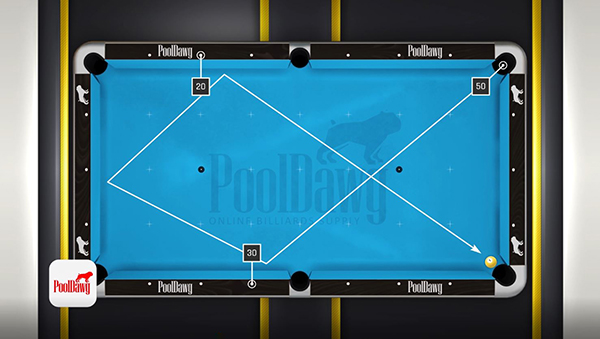So we're going to try that and we're going to see what the table gives us. Always running English. There we go. So we know the table plays quite short, so I didn't miss it by much, but I miss it by at least half a diamond. We know diamond is about 10, so this adjustment amount is a five. What I'm going to do in my formula to adjust it is I'm going to go exactly at the same spot (30), minus five, and that's just to account for the table playing short. Now, if a table plays long, you do plus five. If the table plays normal, you don't do anything, you just keep it there. Now we know the table plays short, so I'm going to do minus five. So 30 minus five, 25. Remember zero, 10, 20, 25. So this is the half out there. And we're going to see what happens.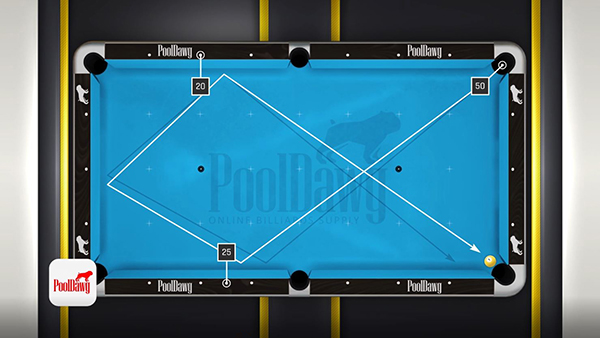Again remember, it's got to be a constant stroke, and it’s good. Okay. So I hit the ball, it didn't quite make it, I didn't set it up perfectly, but we know it's pretty good. So now we have the basics of the system. We know the departure, we know the arrival, and we know the adjustment needed for the table.

## Calculating Three Rail Kicks with the Cue Ball off the Rail

Now that we know the basic premise of the system, it's important to know that this works from everywhere on the table. So I just moved the ball anywhere I want to do so. If I'm here, shooting there or here and the cue ball is still somewhere there, I can still make it. So now let's calculate from the cue ball laying where you just hit.

Ok, we know the arrival already, here at 20. Now the important part is finding my departure. We're going to have to do some guesswork from up there to find where the cue ball lays. We know that if the cue ball was here, our equation would be 50 minus 20 equals 30. The cue ball is not under the line of the cue. Next is 45, 45 minus 20 is 25. The cue ball is still not under. Let's try 40. 40 minus 20 is 20. Look at that, this time it's pretty close. The cue ball is almost under the cue, which is the line we want. Now we know that cue ball is not quite there yet, so I'm going to try another one. Let's do 35. 35 minus 20, it's 15. The cue ball is not there. So obviously the closest point I have is 40.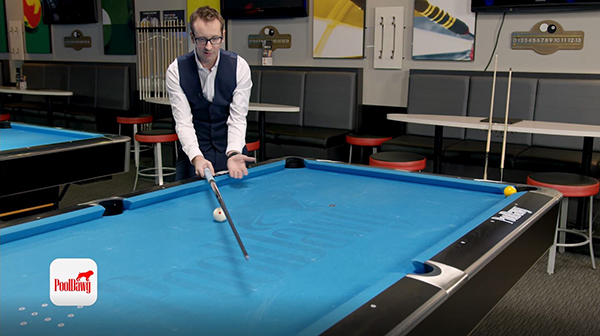So the cue ball is almost in 40, but not quite here. So what I'm going to do is I'm going to guess that it's going to be 42 by moving it this way. Obviously it goes by increments, so it's pretty easy to do. The idea would be 42 minus 20, 22. Again, we know the table plays short by five, 22 minus 5 is 17. So if I aim at 17, which is about right there. I'm a little closer to the rail I'm going to take some off a little bit. I should be right here and make the one ball.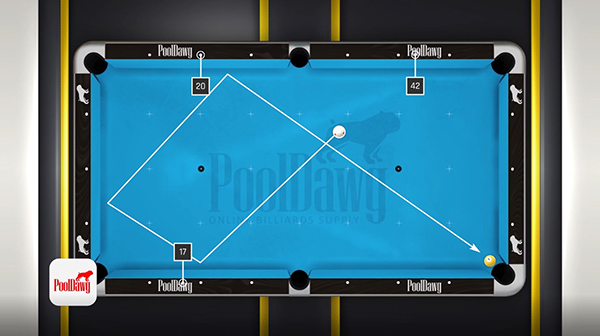There we go. Okay, so that was one example. We're just going to put the cue ball somewhere else. Actually, exactly where it lays out. So let's move it a little bit, right there so it's right on the cross and easy to see. With the one ball there and we're going to do the same thing, another three rail. This ball is in a different position, not out here but more low. So we're going to start from the corner again. So 50, we know the arrival is still 20. 50 minus 20 is 30. It’s not on that line, so we're going to move to here to this diamond. 60, right? 60 minus 20 is 40. Look at that. If I imagine the diamond, which would be right here in the pocket, my cue ball is nearly there. I mean, it's close to perfection so we could go ahead and call it 60.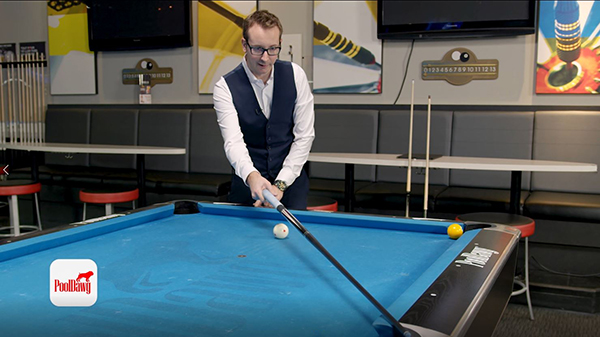Now I know the table plays short, so I'm going to remove five. Let's do this: 60 minus 20 is 40, 40 minus five is 35. That means if I aim the 45, which is right here, I should be able to make the one ball. Now, in this case, it's pretty tricky because the corner of the side pocket is right in my way, which would be right there. We will probably need to aim a little higher and hope for the best.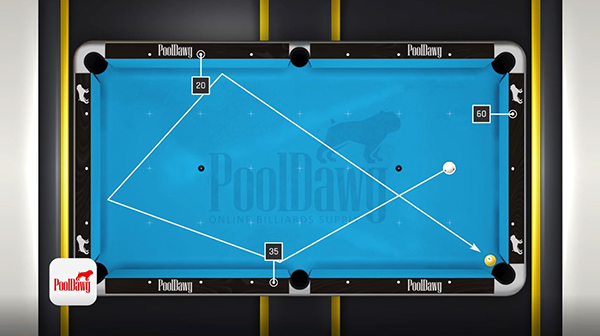There we go. That is how you find where your cue ball is and calculate the exact point needed to land on the one ball.

Now that we figu#aa182c out the departure and where the cue ball can be moved, the object ball is also going to be in a different position every time. This is where it gets a little tricky with this system, in my opinion, because there's some set of numbers that you're going to have to remember, and a set of lines that you're going to have to memorize. But again, memorization is going to help your entire game later on too. So again, we're going to start from here. So we know this corner to here is the line zero. This diamond to here is the line 10. And this obviously to here is the 20. So we're looking at here and the one ball is again, pretty much in the 20.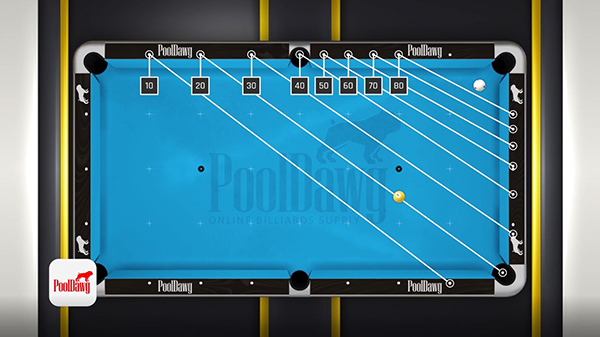So what I'm going to do is take departure, which is 50, 50 minus 20 equals 30. My cue ball is right under the 30 line. Now we know the table plays short so we're going to do 30 minus 5, which is 25. Let's try that.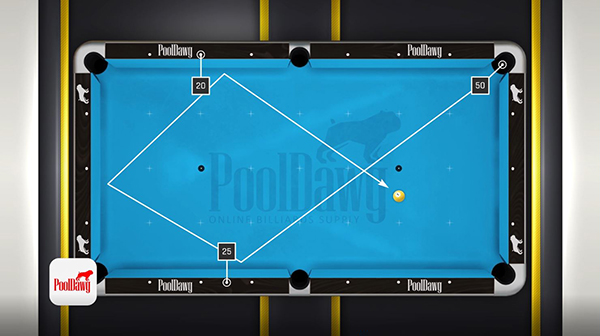And we're spot on. That's the beauty of the system. Everything that's along the line, it doesn't matter if the one ball is here, or here, or here. As long as it's along that line you will be able to hit the ball. Now let's move the ball. Let's pretend this ball is right here. Our cue ball is going to be somewhere on 50. I'm not going to touch that to make it easier. We're going to try to find our object ball.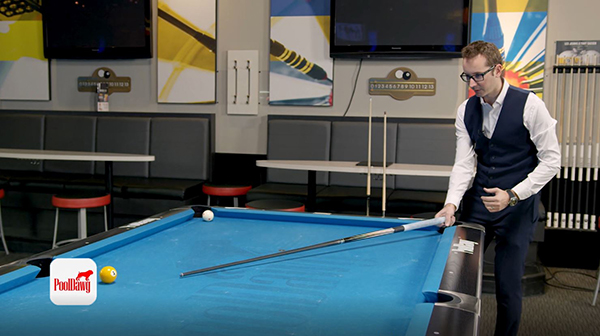So this would be the 20 line. The one is not under the line of the cue and the diamonds. Let's try 30. 30, the object ball is not under there. So let's try 25. 25 would be the half diamond to the half diamond.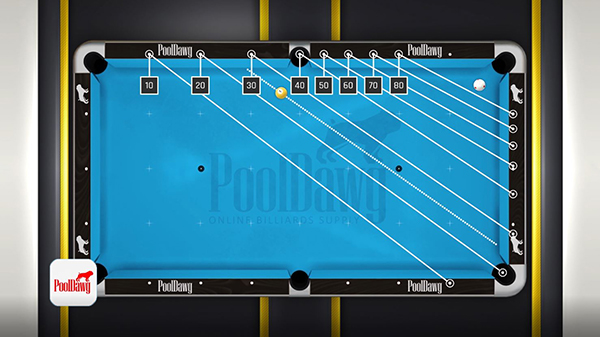Boom, right there. The one ball is under that line of 25. So again, we're going to do departure, 50, minus arrival, 25, equals 25. Then, 25 minus 5 because the table plays short, equals 20. We're right there at the second diamond.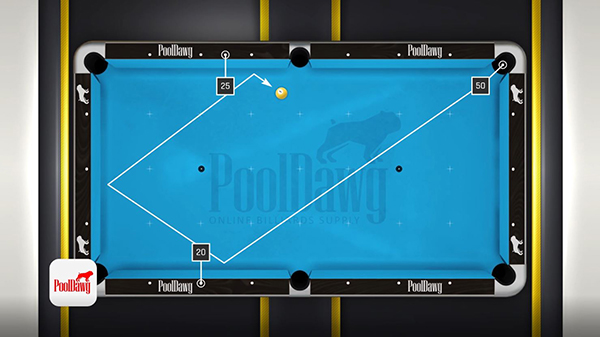There we go. So obviously this wasn't 25, which is not a problem. I can do one last example for the arrival here. We're going to put the ball right here. So that's a very common pool shot when you really can't do much and you have to really try to hit the ball out it to get out of safety or even try to make it. I know a lot of people watch Efren Reyes's videos, this is pretty much the system Efren used. The two rail, the three rail, he uses those things. So that's how it helps you to really progress in your game. All right. So let's look at the system and the math.

Again, we're going to still be in 50, along this line for the departure. The arrival, now so we know it's not 20, because 20 goes here. However, if we look at there, we know it's 10.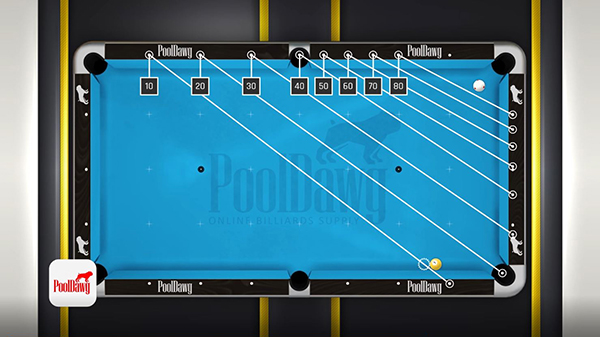So this ball is pretty much on the 10. We're going to do 50 minus 10, which is 40. 40 is the corner. But lucky enough for us, the table plays short, so 40 minus 5 is 35, which is right there in between the half diamonds. So if I am right in front of this, I'm going to go one, two, three, and hopefully touch the one ball and with best of luck, actually make it.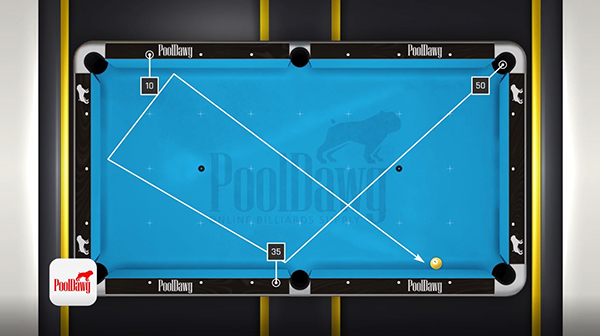I didn't quite make it, but I hit it almost perfect. It really is a very powerful system, and if you know how to calculate, you're going to take a lot of the guesswork out of three-rail kicks. And even if one day you feel like your formulas are off or something, it still gives you a guide and while instinct is important, this really gives you the true line to the perfect shot.

## 3-Rail Examples and Practice Shots

We're back with Valerie here and she's going to help us demonstrate the power of this mathematical system. So before we go any further, it's important to take note of a couple things. This is the exact same example that we did with the visual system. With my mathematical system examples, we are shooting the cue ball this way into that rail, so our departure is here and our arrival there. In this case, it's going to be reversed. So departure is going to be those rails and arrival right here. So first the four ball. The four ball's in the corner, so we know it's at about 20. We know that, and that's fine. Now let's try to find where our departure point is.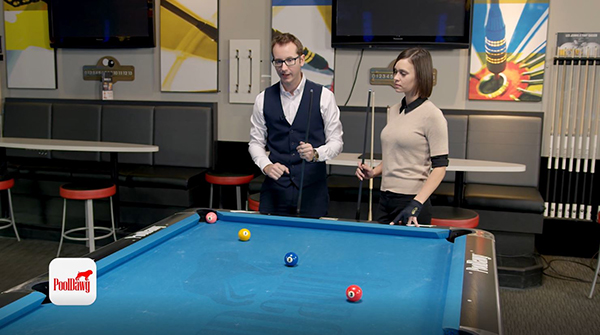So again, we're going to start with the corner here. So this is 50. This is 60. This is 70. This is 80. This is 90. I'm going to take a wild guess and pretend the cue ball is at 70. 70 is that diamond. So I'm going to do 70 minus 20, departure minus arrival, which is 50. So we're going to go there. Aiming is 10, 20, 30, 40, and the half is 50, right here. My cue ball is not in there. In this case, the one ball is the cue ball. So let's try 80. 80's right here, so 80 minus 20 is 60. 10, 20, 30, 40, 50, 60. My cue ball is not quite in their either. If you look at it, it is literally in between 50 and 60. So it's pretty obvious we're going to have to shoot in between. So let's do 75. 75 minus 20, 55. 10, 20, 30, 40, 50, 55. And we are right on it.

Now we know the table plays short so remember to always subtract five. So that puts us at 50. That means if Valerie hits here with running English, she will make the four ball.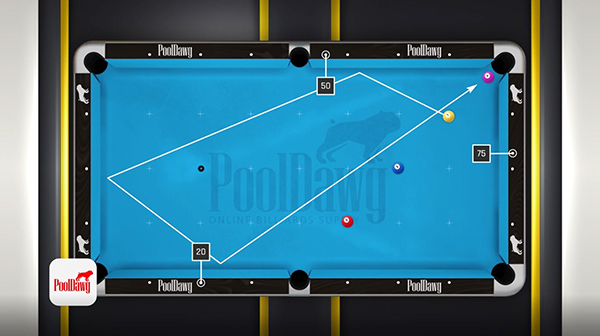There you go, perfect shot. Okay, let's keep going. Now we're going to have to try to find the two ball. So again, our arrival is already known. It's 20. We're going to go here and try to find the departure of the two ball, which is our cue ball this time. We're going to start with 50, 50 minus 20 is 30. The cue ball is not quite in there. Let's do 60. 60 minus 20, is 40. The cue ball is not quite in there. So basically if I go here and there, we know we're right in between, so I'm going to go again and I'm going to guess 55. 55 minus 20, 35. Right there. So we're very close this time. Now we're going to move a little bit and we're going to do maybe 53. 53 minus 20 is 33. We're right on it. Now again, remember the table plays short, so you must subtract 5. 33 minus 5 is 28. If Valerie aims somewhere right here, she should be right on the one ball and make it.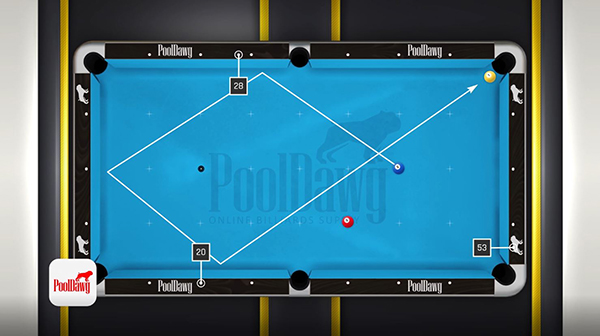Perfect. Let's keep going and do that one last ball, which is the three ball. Again, the three ball is our cue ball. So arrival, still 20, still the pockets. But now this time we need to find a departure, which is higher in the rail. So we know it's not 50. So let's try 45. 45 minus 20 equals 25. I'm going to draw a line from here to the 25, nothing there. Let's do 40. 40 minus 20 equals 20. And we're going to draw a line from 20 there. And the cue ball is literally right under it, which means we are perfectly in there. We know the table plays short by five, so we're going to do 20 minus 5, which is 15. Remember zero, 10, 15. Right here. So if she aims the half diamond there, always running English, we should be right on to make the two.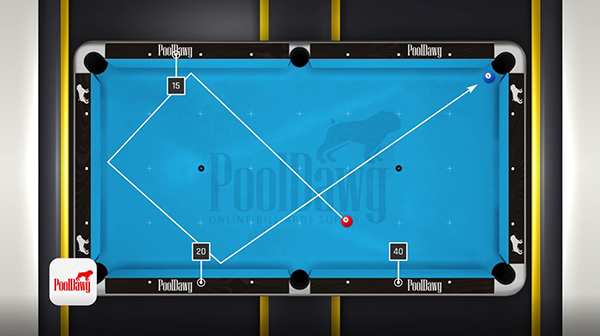There you have it, the same three balls shot in a totally different way than the visual system we talked about at the beginning. You're going to have to decide which one's the best for you. I believe the second one is more accurate, very useful anywhere on the whole table, but the first one might do the job just as well.

This was the three rail kicking system. I hope it's going to help you guys. So just go get to the pool table and practice!

Yeah, it's a very useful system. Takes a little bit to getting used to, especially the second one, but also it's absolutely priceless. As usual, any questions please comment below, let us know what you think. And for all your pool and billiard supplies, please go check out pooldawg.com.